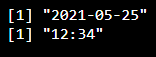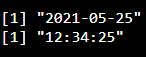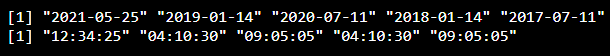# How to separate date and time in R ?

• Last Updated : 29 Jun, 2021

In this article, we are going to separate date and time in R Programming Language.  Date-time is in the format of date and time (YYYY/MM/DD HH:MM:SS- year/month/day Hours:Minute:Seconds).

Extracting date from timestamp: We are going to extract date by using as.Date() function.

Syntax:

as.Date(data)

where data is the time stamp.

Extracting time from the time stamp: We can do this by using as.POSIXct() function. To get a particular time hour format we can use the format() function

Syntax:

format(as.POSIXct(data), format = “%H:%M”)

Where,

• as.POSIXct() is used to extract time from the time stamp
• format is used to get the time format. Ex : hours:Minutes and seconds
• data is the time stamp

Example 1:

## R

 `# create variable with one time stamp ``data =``"2021/05/25 12:34:25"``                            ` `# extract date from the time stamp``print``( ``as.Date``(data))`` ` `# get time from date using format in ``# the form of hours and  minutes ``print``( ``format``(``as.POSIXct``(data), format = ``"%H:%M"``))`

Output:Example 2:

## R

 `# create variable with one time stamp ``data =``"2021/05/25 12:34:25"``                            ` `# extract date from the time stamp``print``( ``as.Date``(data))`` ` ` ` `# get time from date using format in the ``# form of hours ,minutes  and seconds``print``( ``format``(``as.POSIXct``(data), format = ``"%H:%M:%S"``))`

Output:Example 3:

## R

 `# create data  with five time stamps ``data = ``c``(``"2021/05/25 12:34:25"``,``         ``"2019/1/14 04:10:30"``,``         ``"2020/7/11 09:05:05"``,``         ``"2018/1/14 04:10:30"``,``         ``"2017/7/11 09:05:05"``)``                            ` `# extract date from the time stamp``print``( ``as.Date``(data))`` ` `# get time from date using format in the``# form of hours ,minutes  and seconds``print``( ``format``(``as.POSIXct``(data), format = ``"%H:%M:%S"``))`

Output:My Personal Notes arrow_drop_up